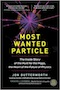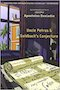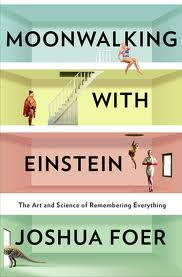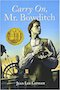# Algebra2 with Trigonometry

### Syllabus:

2018_Syllabus_Alg2H_Block_1_7_9_DrBaharav.pdf(pdf).
Older
2017_Syllabus_Alg2H_Block_3_5_Baharav.pdf(pdf). 2016_Syllabus_Alg2H_Block_3_8_9_Baharav.pdf (H)(pdf).
2016_Syllabus_Alg2_Block_1_Baharav.pdf (Reg)(pdf).
1. Laps1: Laps1.pdf; Laps1_Solution.pdf
2. Laps2: Laps2.pdf; Laps2_Solution.pdf

TOPIC(S) DETAILS
('need to know')
PACKETS and HOMEWORK STUDY GUIDES
and
PAST TESTS
UNIT 1-2:
Algebra review and Equations and Inequalities
Chapters 1,2
Unit1_2_Finals_StudyGuide.pdf Current material:
Honors:
2018-2019
1. Warm-up (Horses_and_Boots), Unit0_Curriculum [pdf], Overview of material for the year, Inquiry-project (Bubble-wrap), Laps1.
2. PEMDAS Warm-up (1..10), PEMDAS worksheet,Syllabus, (start) Lines review (Unit3_HW_Lines Slopes Intro1 Exeter).
2017-2018
1. Warm-up (Horses_and_Boots), Syllabus (Unit0_Curriculum), Overview of material for the year, About me, Inquiry-project (Bubble).
2. Warm-up ( FarmerJohn), PEMDAS (In class), Arithemtic review (HW).
3. Warm-up ( 4Rings), If time left: Solving Equations (left as HW).
4. Warm-up (AbsValue), Solving Equations (going over solutions HW).
5. Inquiry-project: ( Ancestors), Notation (ranges), Exponents, PowerToThePeople. Practice Quiz (HW).
6. Quiz1
7. Warm-up ( PEMDAS_1_to_10), Haiku, Formulas and Exponents (HW).
Challange: E29_Distinctpowers
8. Warm-up ( Crypto999), preparation for (RootOf743332) Jeopardy review (cont), Practice Quiz (HW).
9. Quiz2.
UNIT 3:
Relations, Functions, and Graphs
(aka) Lines
Unit3_Finals_StudyGuide.pdf Current material:
Honors:
Reg:
2018-2019
1. Warm-up ( 4Rings), Continue: Lines review (Unit3_HW_Lines Slopes Intro1 Exeter), Desmos Lines (Match my picture)
2. Lines: Representations ("Unit3_HW_Lines Slopes Representation_In Class WS"), Marble slide lines.a
3. Warmup: Ancestors ,Relations, functions (Unit3_WS_Relations and Functions_0_In Class, Unit3_Relation_Function_1_to_1_In Class), HW: Unit1_HW_ArithmeticReview
4. More lines: "Unit3_HW_Lines Slopes Intro2 Exeter";
2017-2018
1. Inquiry-project: ( Dominos): Table, graph, formula. Inclass worksheet exploration (Unit3_WS_Relations and Functions_0_In Class.pdf): relations-functions (horizontal line test), domain-range.
2. Warmup (-1)^n ;
Finishing WS from last class;
Function composition
Start in class: Relation-functions. Unit3_HW_Relations and Functions_1.pdf
3. Warmup: Hidato;
1-1 function (vertical line test)
"Exploring functions in real world" worksheet (Unit3_HW_Relations and Functions_3_2Functions),
Just start: Operations on functions (composition): Unit3_WS_Relations and Functions_composition.pdf
Maybe also Unit3_HW_Relations and Functions_2.pdf
4. Finish: Operations on functions (composition); Practice quiz.
5. Quiz : Relation, function, 1-1 function ; Domain-Range ; Operations on functions; Composition; Representation: Table, Graph, Algebraic.
6. Inquiry-project: ( Ditch-Diggers): Line, slope, parametrizied. Work sheet (slope etc): Intro to lines.
7. WarmUp: Dog+Cat+Rabbit (Brilliant 100day, day 0), plus a little Explanation on the GridPoint challenge ; Work on 4 common representations of the line equation ; MAYBE: Desmos Match my picture: DesmosLink Worksheet (as HW): Intro to line (2) + absolute-value questions.
8. Marble-slide on desmos!! Fun. DesmosLink
9. Expressing line in different forms. Lines formulas activity: DesmosLink Parallel lines, perpendicular (proof), and some interesting problems.
Prepare a demo: Best deal around!
10. Our own Analytic Geometry DesmosLink Practice test +.
11. Solving practice test + interesting questions.
12. Test
UNIT 4:
Systems of equations
Unit4_Finals_StudyGuide.pdf Current material: Additional material: Honors: Reg:
1. Inquiry-project: Magic Sqaure: System of equations.
We also saw and analyzed the video: Magic Square Party Trick - Numberphile
2. WarmUp: MineSweeper. Worksheet: System of equations-Intro, Unit4_HW_IntroAlgebra_ Exeter.
3. WarmUp: Illuminate (HeptaDecagon challenge). Worksheet: Word problems, Unit4_Z_WordProblems.
4. Worksheet: Word problems (II) - Mixtures review.
Good video on table-method: "Khan academy video: Linear equation word problem: saline"
5. Desmos activity with the worksheet: Lines interpretation of multiple equations + Cramer's rule.
6. Fun with brilliant.com algebra: Algebra: System of unknowns
7. Solving practice test + interesting questions.
8. Test
UNIT 5:
Polynomials and polynomials equations
aka
Factoring
Unit5_Finals_StudyGuide.pdf
Unit5_Finals_JustTheFacts.pdf
Current material:
Honors:
Reg:
1. Inquiry-project: Palindrome 11 ; Minimal factor to 10;
2. Polynomials review + Factoring integers. Unit5_HW_IntroFactoring_ Exeter.
3. Factoring. Unit5_HW_Factoring
4. Challenge solution. Over homework. Factoring (cont.). Unit5_HW_Factoring_II (Cubes, grouping and wrapping up)
5. Unit5_HW_Factoring_Blitz. Solving equations, word problems.
6. Class acitivity: Unit5_factoringPhrase_New. Wrapping up.
7. Class acitivity: Complete the square + practice test.
8. Class acitivity: Self test+grade.
UNIT 6:
Rational expressions
Unit6_Finals_StudyGuide.pdf Worksheets:
Drilling:
• Unit6_Kuta_Reduce_and_Add.pdf [ pdf] ;
• Unit6_Kuta_Long_Division.pdf [ pdf] ;
• Unit6_Kuta_Rational equations.pdf [ pdf] ;
Older stuff:
Honors:
Reg:
1. Intro to unit: Integers and Polynomials. Unit6_WS_I_Intro_Numbers ; Unit6_fractionRealPhrase
3. Long division : Unit6_WS_III_Divide_Long ; Unit6_Kuta_Long_Divisoin
4. Synthetic division : Unit6_WS_III_Divide_Synthetic ;
5. Rational equations: Unit6_WS_IV_Rational_Equations ;
UNIT 7:
Powers, Roots, and Complex Numbers
Unit7_Finals_StudyGuide.pdf Worksheets
Honors: Reg:
1. (warm up the class before: Unit7_KUTA_Properties of Exponents_Easy) ; Intro to the subject: Unit7_WS_Radicals_I ; [HW] Unit7_KUTA_WS_SimplifyingSingleRadical
Fun activity (angles): Desmos Laser
3. Rational exponents: Unit7_WS_Radicals_III ; [HW] Unit7_Kuta_HW_Rational_numbers_as_exponents ;
4. Complex numbers: Unit7_WS_Complex_I ; [HW] Unit7_Kuta_HW_Complex_I.pdf
5. Complex numbers: Unit7_WS_Complex_II ; [HW] Unit7_Kuta_HW_Complex_II.pdf
6. Practice test ; InsightProject_Challenge_Quadratic_1_page.pdf
UNIT 8:
Unit8_Finals_StudyGuide.pdf Current material:
• Unit8_WS_SolvingQuadratic_I (Factoring+completing the square): pdf ; [ docx] ; [ Solution] ;
• Unit8_WS_SolvingQuadratic_II (Quadratic equation/formula): pdf ; [ docx] ; [ Solution] ;
• Unit8_WS_SolvingQuadratic_III (Word problems): pdf ; [ docx] ; [ Solution] ;
• Unit8_HW_Discriminant: pdf ; [ docx] ; [ Solution] ;
• Unit8_WS_Discriminant_solutions: pdf ; [ docx] ; [ Solution] ;
• Drilling:
• Unit8_Kuta_Quadratic Formula.pdf [ pdf] ;
• Unit8_Kuta_The Discriminant.pdf [ pdf] ;
• Unit8_Kuta_Vertex Form of Parabolas.pdf [ pdf] ;
• Unit8_Kuta_Properties of Parabolas.pdf [ pdf] ;
Honors:
Reg:
1. Connecting to Factoring: Unit8_WS_SolvingQuadratic_I
3. ( Jumping to unit-9, and working on graphing quadratics and more)
4. Coming back to this unit:
Short: Discriminant and solutions of quadratic equation. Unit8_WS_Discriminant_solutions (will re-visit after complex numbers) ; HW: Unit8_HW_Discriminant (optional: Kuta 'Quadratic formula' and 'Discriminant' worksheets.)
Desmos: A whole bunch of Parabolas activities: Desmos link
Desmos "Will it hit the hoop" (30mins) Class link
Desmos "Match my parabola" (45mins) Class link
Desmos marbles activity: Parabolas (45mins) Class link
5. Word problems + Pythagoras: "Unit8_WS_SolvingQuadratic_III"
Maybe height-problems "Unit8_HeightproblemsQuadratic equations.pdf"
6. (maybe) Fun activity: 'Golden Gate' bridge parabola."Quad project - Alg II Honors - Task 1.pdf"
7. Practice test. Some resources:
UNIT 9:
Quadratic functions and transformations
Unit9_Finals_StudyGuide.pdf Current material:
Honors:
Reg:
1. Graphing: Graph transformations. "Unit9_MathLab_funcs_lab parent graphs and transformations.pdf" .
2. Graphing quadratics worksheet (f(x) ) ("Unit9_Exploration_WS.pdf")
3. Representation as a(x-h)^2+k ("Unit9_WS_Vertex_form") and transforming among different representations.
4. Graphing: Multiple representations and switching among these."Unit9_FunMultipleGraphs_WS.pdf"
5. (Moved from here to 8) Quadratic equations: Minimum and Maximmum, Spot (the dog). "Unit9_Quadratic equations_Spot_1_3.pdf"
UNIT 10:
Conic sections
Did not do (was not in plan)
UNIT 11: Polynomials
Unit11_Finals_StudyGuide.pdf Current material:
Previous material:
Honors:
Reg:
1. Introduction to polynomials, End behavior.
2. Factors
3. Factors (again) + laps
4. Theorems: remainder, rational roots.
5. Practice test, Challenge (partial graph)
6. Unit test
UNIT 12: Inverse functions, Exponents, and Logarithms
Unit12_Finals_StudyGuide.pdf
1. Unit12_WS_Inverse_I_IntroAndTerms ; Unit12_inverse_ZZ_inverseWorksheet_Reorg_newer
2. Unit12_inverse_practice inverses graph and alg a ;
UNIT 14: Sequences and Series
Unit14_Finals_StudyGuide.pdf
• Unit14_WS_Sequences_I: pdf ; [ docx] ; [ Solution] ;
• Unit14_WS_Arithmetic_II: pdf ; [ docx] ; [ Solution] ;
• Unit14_WS_Geometric_III: pdf ; [ docx] ; [ Solution] ;
• Unit14_WS_Geometric_Infinite_IV: pdf ; [ docx] ; [ Solution] ;
• Drilling:
• Unit14_Kuta_Introduction to Sequences.pdf [ pdf] ;
• Unit14_Kuta_Arithmetic_seq_sum.pdf [ pdf] ;
• Unit14_Kuta_Geometric_sequences_and_series.pdf [ pdf] ;
• Unit14_Kuta_Infinite_geometric_sum.pdf [ pdf] ;
Honors:
1. Intro to sequences: Unit14_WS_Sequences_I.
2. Arithmetic: Unit14_WS_Arithmetic_II.
3. Geometric: Unit14_WS_Geometric_III.
4. Geometric infinite: Unit14_WS_Geometric_Infinite_IV.
UNIT 18: Trigonometry of right triangle
Finals: Comprehensive test
Honors: Reg:

* Credit to : http://www.carlisleschools.org/webpages/wolfer
```		Mrs. Wolfe
Carlisle High School, M16
623 West Penn Street
Carlisle, PA 17013
McGowan Building, Room M16
```
** Credit to : Chicago teacher union quest center www.sharemylesson.com/ctu
*** Credit to : TPT (ToDo: Add details)

=================================="Most wanted particle" ,by Jon Butterworth. The hunt for the Higgs Boson, which ended successfully (and with a Nobel laureate in 2013). An amazing inside story about the most basic particles. Higgs boson is also called the 'God particle', and it is  the one who can create mass from energy. Highly recommend, but it would definitely help if you did take a course in quantum mechanics etc. June-17-2017."Uncle Petros and Goldbach's Conjecture: A Novel of Mathematical Obsession" ,by Apostolos Doxiadis. The beauty of pure mathemtics, and pursuing interest in a susbject for it's own aestethics and beauity. January-01-2017."The Martian" ,by Andy Weir. Fiction. NASA astrounaut stranded on Mars. Interesting 'action/thriller' kind of book. A somewhat interesting question: What is the value of human life? (and i mean in dollars!). OR maybe it's priceless? January-26-2016."Moonwalking with Einstein", by Joshua Foer. (Boomook as well) Non fiction. The subtitle is: "The art and Science of Remembering Everything". A year long journey into the art of memorizing stuff. With Google and computers at our fingertips, relying on external-memory is the norm. Is there no value to actual memorization? Very readable and enjoyable. Recommend! Oct-06-2013."Carry On, Mr. Bowditch", by Jean Lee Latham. Motivating book on the development of maritime navigation. Not one formula in there! 2011.

Questions/Comments: Zbaharav at Kehillah dot Org or Zachi at Baharav dot Org .